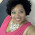## Monday, 7 October 2013

### Paired Student's t-test

The paired Student's t-test is used when two datasets have the same number of samples, and are matched in some way.  For example, the first dataset could be the weight of a group of dieters before they embark upon a particular diet regime, while the second dataset would be the weight of the same group of volunteers two months into their diet program. Sample 1 of the first dataset matches sample 1 of the second dataset, and so on i.e. sample $k$ of the first group matches sample $k$ of the second dataset.

For this test to yield accurate results, the samples must have come from a Normal distribution.  A more robust equivalent test that does not require this assumption is the non-parametric Wilcoxon Signed-Rank test (see blog posting dated 03/09/2013). Nevertheless, if the samples do indeed come from a Normal distribution, this parametric test will be more sensitive.

Let $x_1(n)$ denote the samples for the first dataset, and $x_2(n)$ denote the samples for the second dataset, for index $n$ ranging from $1$ to $N$ inclusive, i.e. the datasets comprise $N$ samples.

We find the sample difference $d(n)=x_1(n)-x_2(n)$, and calculate the mean of the differences:-
$\Large \mu=\frac{1}{N}\sum_{n=1}^Nd(n)$

Next we calculate the Standard Deviation of the differences:-
$\Large \sigma=\sqrt{\frac{1}{N-1}\sum_{n=1}^N(d(n)-\mu)^2}$

Once we have the mean and standard deviation of the sample differences, we are in a position to calculate the $t$ value:-
$\Large t=\frac{\mu}{\sigma/\sqrt{N}}$

After obtaining the $t$ value, we can calculate the $p$ value, which is the probability that the observed sample differences (or values of differences that are even greater) arise purely from chance. Assuming we have a two-tailed scenario (in other words the difference between the two matched groups could be either positive or negative), we have

If $t>0$
$p=2\times(1-student\_t\_cdf(t,N-1))$

If $t\leq 0$
$p=2\times student\_t\_cdf(t,N-1)$

where $student\_t\_cdf(t,N-1)$ is the Cumulative Distribution Function (CDF)  of the Student-t distribution with degree of freedom $N-1$, and integrating from $-\infty$ to $t$.

The $p$ value can be calculated using software packages (which implement some numerical method of evaluating the CDF of the Student-t distribution), using tables, or using the online Student-t CDF calculator that I have posted here. If you do own an iPhone, you can always download my free app SciStatCalc, which can be used to calculate both the CDF and the quantile function of 19 distributions (including, of course, the Student t distribution!).

The smaller the value of $p$, the less likely the difference is due to chance alone (which is our Null Hypothesis where we assume the matched groups are similar save for any random fluctuations), and the more significant the result is. We can arbitrarily assign a significance value of 0.05 (5%), say, and if $p$ is below this value, we deem the result significant and reject the Null Hypothesis.

We can also find, say, the 95% confidence interval for the sample differences using the quantile function of the Student t distribution (also known as the inverse CDF).
The lower limit is
$\large low\_lim=\mu-(\frac{\sigma}{\sqrt{N}}\times(inv\_t\_cdf(0.975,N-1)))$

The upper limit is
$\large upper\_lim=\mu+(\frac{\sigma}{\sqrt{N}}\times(inv\_t\_cdf(0.975,N-1)))$
As we are using a two-tailed test, the area under the density at each end of the distribution will be half the 5% (i.e. 0.025), so that the CDF upper limit is 1-0.025=0.975.

An online Paired Student-t Calculator can be found on this blog in here.

#### 1 comment:

1.Good luck to anyone reading this true life story of mine, I Was Diagnosed With type 2 Herpes Virus Last year, And I Was Looking For Solution To Be Cured Luckily I Saw Testimonies On How Dr OYAGU Cure Herpes Virus I Decided To Contact Dr OYAGU I Contacted Him He Prepared A Herbal Medicine Portion And Sent It To Me, I Started The Herbal Medicine For My Health. He Gave Me Step By Step Instructions On How To Apply It, When I Applied It As Instructed, I Was Cured Of This Deadly Herpes Within 2 weeks, I Am Now Herpes Negative. My Brother And Sister I No That There Are So Many People That Have The Same Herpes Virus Please contact Dr OYAGU To Help You Too, And Help Me To Thank Dr OYAGU For Cure Me, I’m Cured By Dr. OYAGU Herbal Medicine, His Contact Email:oyaguherbalhome@gmail.com or visit his website https://oyaguspellcaster.wixsite.com/oyaguherbalhome Or Cell Whatsapp Number +2348101755322 thank you